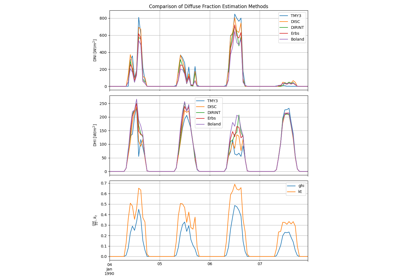pvlib.irradiance.dirint(ghi, solar_zenith, times, pressure=101325.0, use_delta_kt_prime=True, temp_dew=None, min_cos_zenith=0.065, max_zenith=87)[source]#

Determine DNI from GHI using the DIRINT modification of the DISC model.

Implements the modified DISC model known as “DIRINT” introduced in 1. DIRINT predicts direct normal irradiance (DNI) from measured global horizontal irradiance (GHI). DIRINT improves upon the DISC model by using time-series GHI data and dew point temperature information. The effectiveness of the DIRINT model improves with each piece of information provided.

The pvlib implementation limits the clearness index to 1.

Parameters
• ghi (array-like) – Global horizontal irradiance in W/m^2.

• solar_zenith (array-like) – True (not refraction-corrected) solar_zenith angles in decimal degrees.

• times (DatetimeIndex) –

• pressure (float or array-like, default 101325.0) – The site pressure in Pascal. Pressure may be measured or an average pressure may be calculated from site altitude.

• use_delta_kt_prime (bool, default True) – If True, indicates that the stability index delta_kt_prime is included in the model. The stability index adjusts the estimated DNI in response to dynamics in the time series of GHI. It is recommended that delta_kt_prime is not used if the time between GHI points is 1.5 hours or greater. If use_delta_kt_prime=True, input data must be Series.

• temp_dew (None, float, or array-like, default None) – Surface dew point temperatures, in degrees C. Values of temp_dew may be numeric or NaN. Any single time period point with a temp_dew=NaN does not have dew point improvements applied. If temp_dew is not provided, then dew point improvements are not applied.

• min_cos_zenith (numeric, default 0.065) – Minimum value of cos(zenith) to allow when calculating global clearness index kt. Equivalent to zenith = 86.273 degrees.

• max_zenith (numeric, default 87) – Maximum value of zenith to allow in DNI calculation. DNI will be set to 0 for times with zenith values greater than max_zenith.

Returns

dni (array-like) – The modeled direct normal irradiance in W/m^2 provided by the DIRINT model.

Notes

DIRINT model requires time series data (ie. one of the inputs must be a vector of length > 2).

References

1

Perez, R., P. Ineichen, E. Maxwell, R. Seals and A. Zelenka, (1992). “Dynamic Global-to-Direct Irradiance Conversion Models”. ASHRAE Transactions-Research Series, pp. 354-369

2

Maxwell, E. L., “A Quasi-Physical Model for Converting Hourly Global Horizontal to Direct Normal Insolation”, Technical Report No. SERI/TR-215-3087, Golden, CO: Solar Energy Research Institute, 1987.

## Examples using `pvlib.irradiance.dirint`#Diffuse Fraction Estimation

Diffuse Fraction Estimation